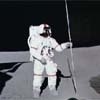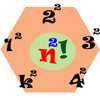#### You may also like### Upsetting Pitagoras

Find the smallest integer solution to the equation 1/x^2 + 1/y^2 = 1/z^2### Lunar Leaper

Gravity on the Moon is about 1/6th that on the Earth. A pole-vaulter 2 metres tall can clear a 5 metres pole on the Earth. How high a pole could he clear on the Moon?### Seriesly

Prove that k.k! = (k+1)! - k! and sum the series 1.1! + 2.2! + 3.3! +...+n.n!

# Universal Time, Mass, Length

##### Age 16 to 18 ShortChallenge Level

We have had two very impressive, and beautifully presented, solutions to this problems, from Laura , from The Henrietta Barnett School and Steven , from City of Sunderland College.

We need the values of $1\,\textrm{cm}$, $1\,\textrm{s}$, $1\, \textrm{J}$ and $1\, \textrm{K}$ in terms of imperial units. These are
$$1\,\textrm{cm} = \frac{1}{30.48}\textrm{ft}\quad1\,\textrm{g} = \frac{2.2}{1000}\textrm{lb}\quad 1\,\textrm{J} = \frac{1}{4.184}\, \textrm{cal}\quad 1\,\textrm{K} = 1.8^\circ\, \textrm{F}$$
These values can now be substituted into the numerical expressions for $G, h, c, k$. For Newton's constant we have
$$\begin{eqnarray} G& =& 6.674 \times 10^{-8} \textrm{cm}^3 \textrm{g}^{-1} \textrm{s}^{-2}\\ &=& 6.674 \times 10^{-8} \left(\frac{1}{30.48}\textrm{ft}\right)^3\left(\frac{2.2}{1000}\textrm{lb}\right)^{-1}s^{-2}\\ &=& \frac{6.674\times 10^{-8}\times 1000}{30.48^3\times 2.2}\textrm{ft}^3 \textrm{lb}^{-1} \textrm{s}^{-2}\\ &=& 1.071\times 10^{-9}\textrm{ft}^3\textrm{lb}^{-1}\textrm{s}^{-2} \end{eqnarray}$$
In a similar way, we can find that
$$c = 9.836\times 10^8 \textrm{ft}\, \textrm{s}^{-1}\quad \quad h = 2.496\times 10^{-33} \textrm{lb}\, \textrm{ft}^2 \textrm{s}^{-1} \quad\quad k = 1.83\times 10^{-24}\textrm{cal} ^\circ \textrm{F}$$

For the second part of the problem, Steven noted the following

In a system of units where $G, c, h, k$ are numerically equal to 1, let $L, M, T$ be the units of length, mass and time respectively, so that
$$\begin{eqnarray} G&=& 1 L^3M^{-1}T^{-2}\\ c&=& 1 LT^{-1}\\ h&=& 1 ML^2 T^{-1} \end{eqnarray}$$
We now need to solve for $M, L, T$ in terms of $c, G, h$

After some rearranging, Steven found out that

$$M=\sqrt{\frac{ch}{G}}\quad\quad L=\sqrt{\frac{Gh}{c^3}}\quad\quad T=\sqrt{\frac{Gh}{c^5}}$$

Putting in the numerical values given in the question yielded

$$M = 2.176\times 10^{-5}\textrm{g}\quad\quad L = 1.616\times 10^{-33}\textrm{cm}\quad\quad T = 5.389\times 10^{-44}\textrm{s}$$

Steven finally concluded with a clever extension in which he found a natural temperature scale for the universe as follows:

From the equation Work = Force $\times$ Distance it can be seen that $J = \textrm{Nm}$, and from the equation Force = Mass $\times$ Acceleration we have that $\textrm{N} = \textrm{kg}\, \textrm{m}\, \textrm{s}^{-2}$. So, the units of the Bolzmann constant are $\textrm{kg}\,\textrm{m}^2\textrm{s}^{-2}\textrm{K}^{-1}$.
So, as before, supposing the $\theta$ is the natural temperature scale for the universe.
$$k = 1 ML^2T^{-2}\theta^{-1}\;.$$
Solving as before gives
$$\theta = \sqrt{\frac{c^5h}{Gk^2}}\,.$$
The Boltzmann constant must be converted to have the same units as the other constants before evaluating this result. Putting in the numbers gives
$$k = 1.38\times10^{-16}\textrm{g}\, \textrm{cm}^2 \textrm{s}^{-2}\textrm{K}^{-1}\;.$$
We can now substitute this value into the expression for $\theta$ to give
$$\theta = 1.42\times 10^{32}\textrm{K}\;.$$